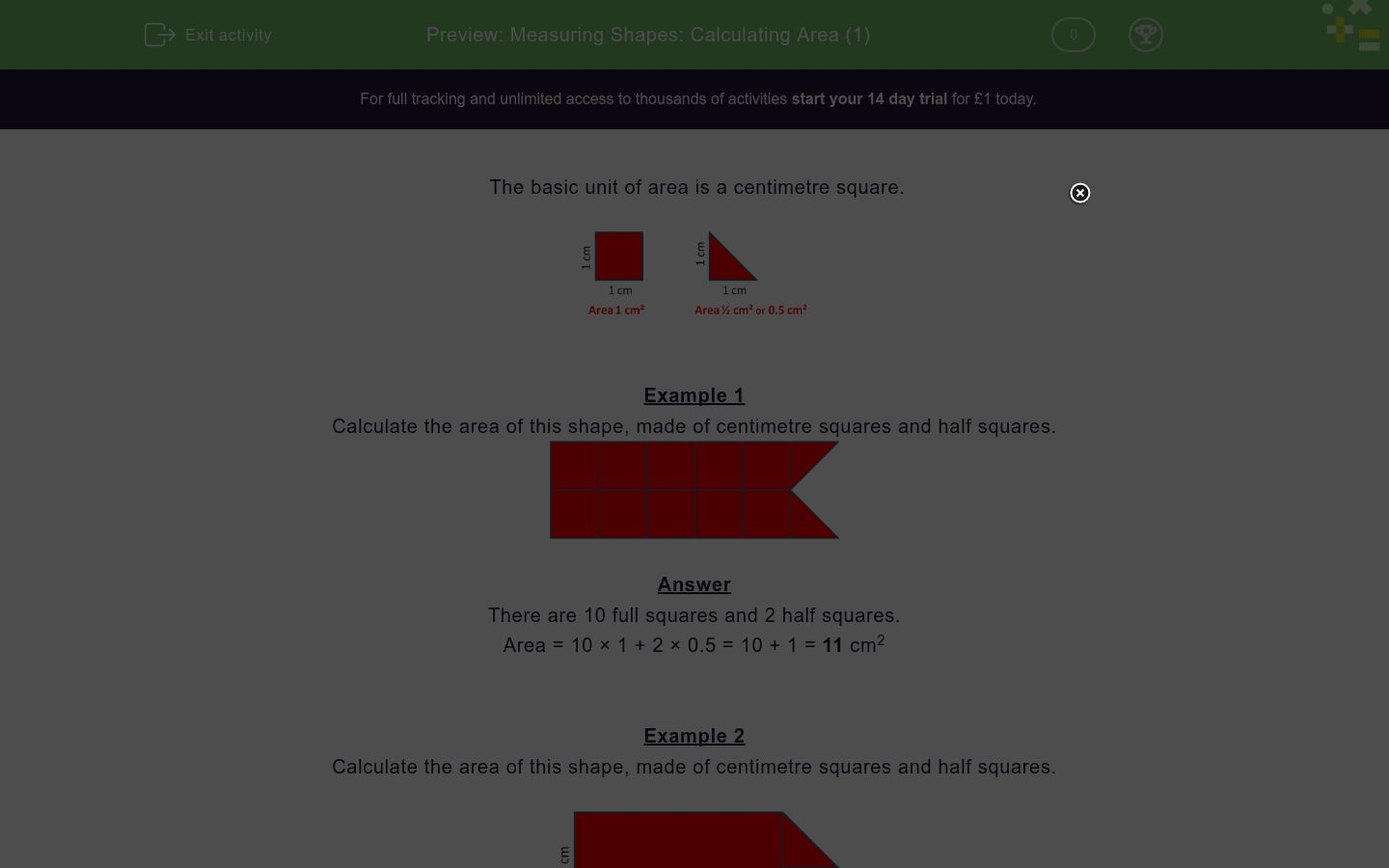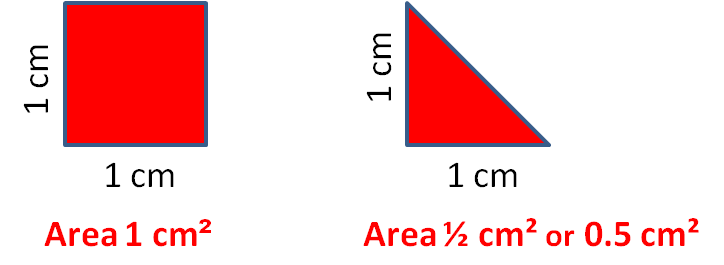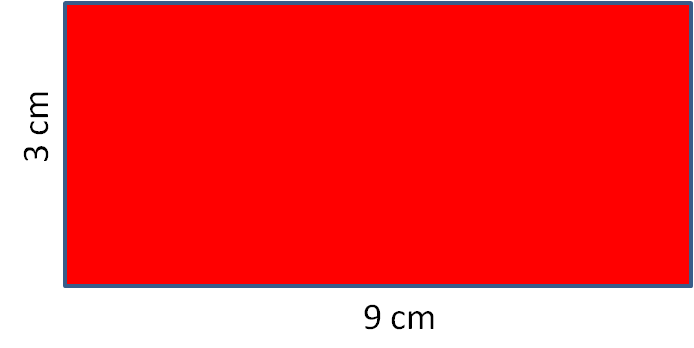# Measuring Shapes: Calculating Area (1)

In this worksheet, students work out the areas of simple shapes made of squares and half squares.Key stage:  KS 2

Curriculum topic:   Measurement

Curriculum subtopic:   Calculate Areas

Difficulty level:### QUESTION 1 of 10

The basic unit of area is a centimetre square.Example 1

Calculate the area of this shape, made of centimetre squares and half squares.There are 10 full squares and 2 half squares.

Area = 10 × 1 + 2 × 0.5 = 10 + 1 = 11 cm2

Example 2

Calculate the area of this shape, made of centimetre squares and half squares.The area of a rectangle = length × width

Area of rectangle = 3 × 2 = 6 cm2

Area of triangle = 0.5 cm2

Area of shape = 6 + 0.5 = 6.5 cm2

Want to understand this further and learn how this links to other topics in maths?
Why not watch this video?

﻿Calculate the area of this shape, made of centimetre squares and half squares.﻿Calculate the area of this shape, made of centimetre squares and half squares.﻿Calculate the area of this shape, made of centimetre squares and half squares.﻿Calculate the area of this shape, made of centimetre squares and half squares.﻿Calculate the area of this shape, made of centimetre squares and half squares.﻿Calculate the area of this shape, made of centimetre squares and half squares.﻿Calculate the area of this shape, made of centimetre squares and half squares.﻿Calculate the area of this shape, made of centimetre squares.﻿Calculate the area of this shape, made of centimetre squares.﻿Calculate the area of this shape, made of centimetre squares and half squares.• Question 1

﻿Calculate the area of this shape, made of centimetre squares and half squares.7
EDDIE SAYS
6 squares + 2 x 0.5 triangles = 7
• Question 2

﻿Calculate the area of this shape, made of centimetre squares and half squares.8
EDDIE SAYS
6 squares + 4 x 0.5 triangles = 8
• Question 3

﻿Calculate the area of this shape, made of centimetre squares and half squares.6.5
EDDIE SAYS
5 squares + 3 x 0.5 triangles = 6.5
• Question 4

﻿Calculate the area of this shape, made of centimetre squares and half squares.7.5
EDDIE SAYS
5 squares + 5 x 0.5 triangles = 7.5
• Question 5

﻿Calculate the area of this shape, made of centimetre squares and half squares.8.5
EDDIE SAYS
5 squares + 7 x 0.5 = 8.5
• Question 6

﻿Calculate the area of this shape, made of centimetre squares and half squares.10
EDDIE SAYS
5 squares + 10 x 0.5 triangles = 10
• Question 7

﻿Calculate the area of this shape, made of centimetre squares and half squares.8.5
EDDIE SAYS
Area = length x width A = 4 x 2 = 8 8 + 0.5 = 8.5
• Question 8

﻿Calculate the area of this shape, made of centimetre squares.27
EDDIE SAYS
Area = length x width A = 9 x 3 = 27
• Question 9

﻿Calculate the area of this shape, made of centimetre squares.35
EDDIE SAYS
Area = length x width A = 7 x 5 = 35
• Question 10

﻿Calculate the area of this shape, made of centimetre squares and half squares.9
EDDIE SAYS
Area = length x width A = 4 x 2 = 8 8 + 2 x 0.5 = 9
---- OR ----

Sign up for a £1 trial so you can track and measure your child's progress on this activity.

### What is EdPlace?

We're your National Curriculum aligned online education content provider helping each child succeed in English, maths and science from year 1 to GCSE. With an EdPlace account you’ll be able to track and measure progress, helping each child achieve their best. We build confidence and attainment by personalising each child’s learning at a level that suits them.

Get started